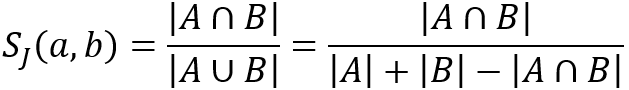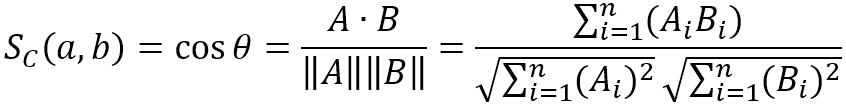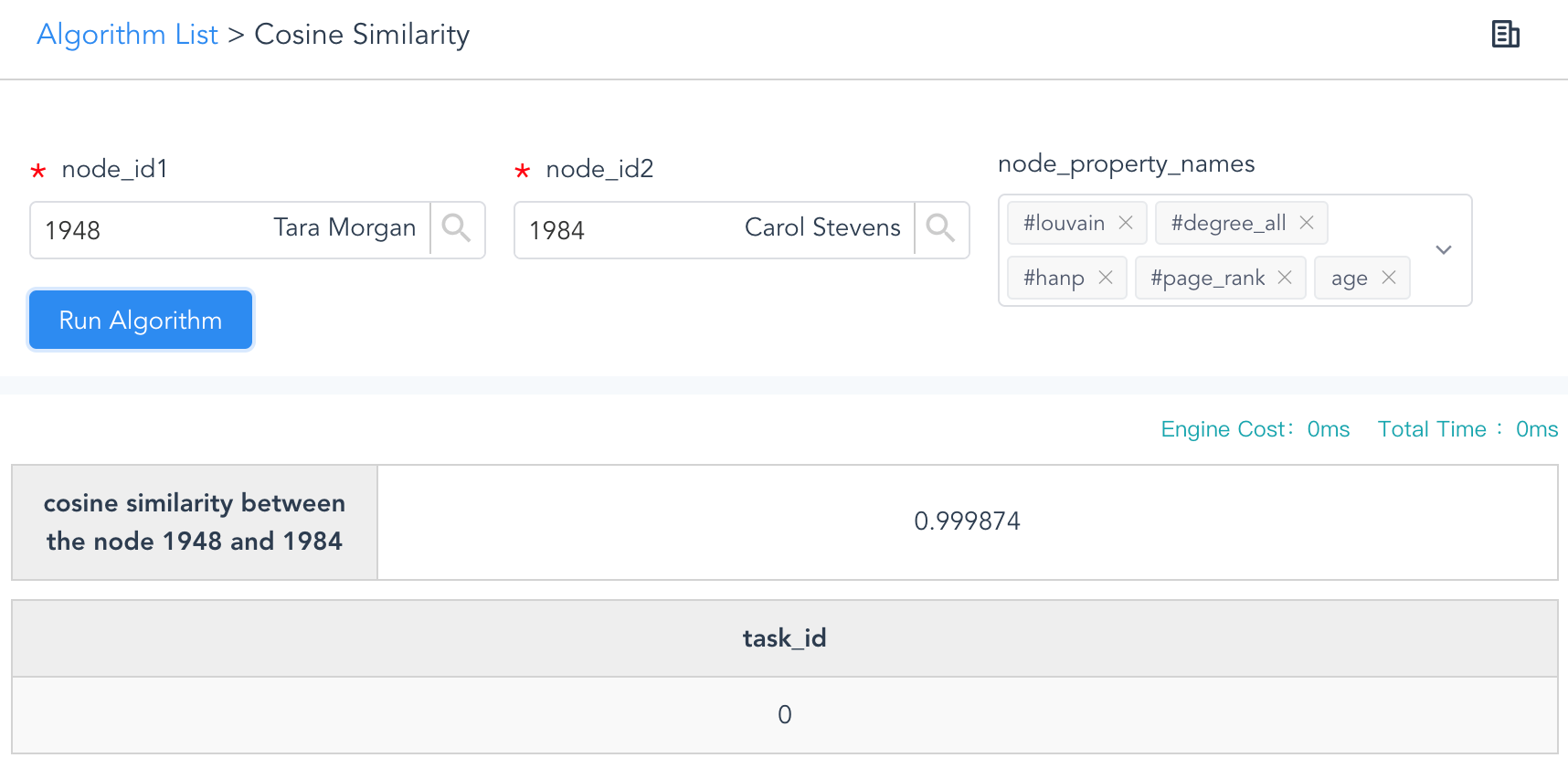# Change Nickname

Current Nickname:
Search
v2.x
v2.x

# Similarity

### `algo(jaccard)`

Basic     Real-time

Jaccard Similarity is also known as Intersection over Union. It basically measures the similarity between two finite samples sets, and is defined as the size of the intersection divided by the size of the union of these two sample sets. Obviously, this coefficient is a value between 0 and 1, the larger the coefficient, the higher the similarity.

Jaccard Similarity by Ulitpa Graph calculates the similarty between two nodes in terms of their neighborhood. As expressed below, A and B represent the neighbor sets of node a and node b respectively (deduplicated and excluding the subject node a and b), and the similarity is calculated as the nubmer of their common neighbors divided by the nubmer of all their neighbors.Configuration items for Jaccard Similarity operation:

Item Data Type Specification Description
`<ids1>` []int Ultipa ID ID of node a, input multiple nodes for a batch computing
`<ids2>` []int Ultipa ID (Optional) ID of node b, input multiple nodes for a batch computing;
nodes from `<ids1>` and `<ids2>` will be paired and calculated, or nodes from `<ids1>` will be paired with any different nodes from the graph if not configured
`<limit>` int >0; -1 `<ids2>` configured: The maximum number of results to return; -1: return all the results
`<ids2>` not configured: The maximum number of similar nodes to return for each node in group a; -1: return all the similar nodes for each node in group a
`<order>` string 'ASC' or 'DESC' (Optional) To arrange the results in ascending or descending order, or leave them un-ordered if not configured

Calculation results:

Item Data Type Range
the Jaccard similarity of node pairs float [0, 1]

Validity of `write_back()`:

Not supported.

Example 1: Calculate Jaccard Similarity between each pair of nodes from [1,2,3] and [4,5,6], return the top 5 results

``````algo(jaccard).params({ ids1: [1,2,3], ids2: [4,5,6], limit: 5, order: 'DESC' })
``````

Example 2: For each nodes in [1,2,3], calculate the top 3 most similar nodes

``````algo(jaccard).params({ ids1: [1,2,3], limit: 3 })
``````

### `algo(cosine_similarity)`

Basic     Real-time

Cosine similarity, by definition, is a measure of similarity between two non-zero vectors of inner product space. In the context of a graph data set, a non-zero vector is a node represented by property values. Given two nodes a and b represented by properties (a1,a2,a3...) and (b1,b2,b3...), their Cosine similarity is:The result of computed cosine similarity ranges from 0 to 1, 1 means 100% similar, 0 means no-similarity at all.

Configuration items for Cosine Similarity operation:

Item Data Type Specification Description
`<node_id1>` int Ultipa ID The node1
`<node_id2>` int Ultipa ID The node2
`<node_property_names>` []string comma (,) separated, at least two node properties of numeric type The node properties (must be LTE first) to be used for calculation

Calculation results:

Item Data Type Range
the Cosine similarity of the node pair float [0, 1]

Validity of `write_back()`:

Not supported.

Example: By using the 'salary' and 'age' properties of node 12 and node 21, calculate their cosine similarity:

``````algo(cosine_similarity).params({
node_id1: 12,
node_id2: 21,
node_property_names: ["salary", "age"]
})
``````

To launch Cosine Similarity algorithm with Ultipa Manager, you simply need to go to Algos module and enter the ID of two nodes plus the node properties to be used for computing, the instant results are shown in the below screenshot:Figure: Cosine Similarity (Ultipa Manager)